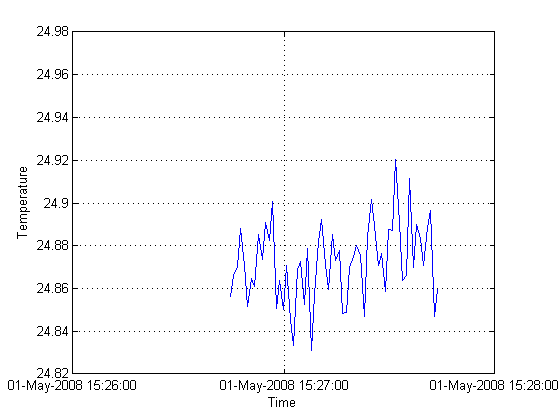# Converting National Instruments LVM Timestamps to Excel (UPDATED: and Matlab)

A few days ago, I had a student looking into what would be required to periodically log some temperatures and pressures from a long-running furnace experiment, so that he doesn’t have to babysit it and come back every 30 minutes to record his data. We borrowed a National Instruments USB-6008 data acquisition device and downloaded NI SignalExpress LE to try some things out.

There wasn’t much of a problem with actually capturing the data, but the timestamps (in column 1) were odd-looking:

```LabVIEW Measurement
Writer_Version	0.92
Separator	Tab
X_Columns	One
Time_Pref	Absolute
Date	2008/04/11
Time	16:24:24.354863

Channels	1
Samples	100
Date	2008/04/11
Time	16:22:45.354864
X_Dimension	Time
X0	0.0000000000000000E+0
Delta_X	1.000000
X_Value	Voltage - Dev1_ai0	Comment
3.29079376535486410E+9	2.64872102540972910E+0
3.29079376635486410E+9	2.64872102540972910E+0
3.29079376735486410E+9	2.64872102540972910E+0
3.29079376835486410E+9	2.64872102540972910E+0
3.29079376935486410E+9	2.65280301912685700E+0
3.29079377035486410E+9	2.65280301912685700E+0
```

There may be a simple way to reformat them inside SignalExpress LE, but it wasn’t obvious enough when we looked. So here’s one solution.

The primary difference between National Instruments’ timestamp format and Excel’s is that NI counts a real-valued number of seconds since January 1, 1904, while Excel counts a real-valued number of days since January 1, 1900. So
the formula (A6/86400)+365*4+2 (convert seconds into days, add four years plus two leap days for 1900 and 1904) will convert NI’s timestamp value of 3290793765.35486 in cell A6 into an Excel equivalent 39549.8908, or April 11, 2008.

But that’s not quite enough. The test run we made was at 4:22 PM, and that’s nowhere near 89% of a full day. Sure enough, putting 39549.8908 into a time format gave us 9:22:45 PM, a full five hours ahead of local time. So there’s a timezone shift in there, too, and we’re currently on GMT-5. So strip off the fractional part of the Excel timestamp, offset it by 5/24, and convert it back into a time. The final resulting spreadsheet in tabular form:

 A B C D E F G H I J 5 Original LVM Time Original LVM Voltage Convert Secs to Days Offset by 4 Years (plus 2 leap days) Final Date Time (before timezone offset) Decimal part of Timestamp Offset for Timezone Final Time Final Date/Time 6 3290793765.35486 2.64872102540972 =A6/86400 =C6+365*4+2 =D6 =D6 =D6-ROUNDDOWN(D6,0) =G6+\$C\$3/24 =H6 =ROUNDDOWN(E6,0)+H6

where cell C3 contained a -5 for our timezone shift from GMT. Columns E, F, I, and J were all in date, time, or date/time format as needed. The result in cell J6 could be cut down to `=(A6/86400+365*4+2)+\$C\$3/24` if you’re in a rush to just convert it.

There may be a math or other error that’s been compensated for somewhere in here, since I’m not 100% positive about the 2 leap days. But it does equal out down to the second.

Matlab has another time format altogether — it uses a real-valued number of days since the zeroth of January, year 0000, whatever that means:

```>> datestr(0,0)

ans =

00-Jan-0000 00:00:00

>> datestr(1,0)

ans =

01-Jan-0000 00:00:00

>> datestr(1/86400,0)

ans =

00-Jan-0000 00:00:01
```

Since that goes back far enough to include the current Gregorian calendar, Julian calendar, and possibly even the pre-Julian Roman calendar standards, the conversion equation is a bit more obtuse (in particular, the 97 day offset was found entirely by trial and error):

```tzoffset=-5;
time=data(:,1);
temperature=data(:,2);
time=time/86400+365*1905+97+tzoffset/24;
plot(time,temperature);
datetick('x',0);
grid on;
xlabel('Time');
ylabel('Temperature');
```

resulting in the correct plot for the brief experiment:## Join the Conversation

1.1 Comment

1.Tom says:

The Excel formula can be simplified to =DATE(1904,1,1)+A6/86400 for GMT.